### The Basic Model

Suppose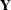represents the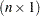vector of observed data and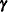is a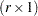vector of random effects. Models fit by the GLIMMIX procedure assume that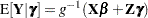where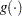is a differentiable monotonic link function and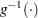is its inverse. The matrixis an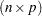matrix of rank, and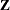is an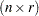design matrix for the random effects. The random effects are assumed to be normally distributed with mean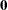and variance matrix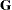.

The GLMM contains a linear mixed model inside the inverse link function. This model component is referred to as the linear predictor,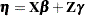The variance of the observations, conditional on the random effects, is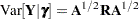The matrix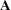is a diagonal matrix and contains the variance functions of the model. The variance function expresses the variance of a response as a function of the mean. The GLIMMIX procedure determines the variance function from the DIST= option in the MODEL statement or from the user-supplied variance function (see the section Implied Variance Functions). The matrix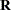is a variance matrix specified by the user through the RANDOM statement. If the conditional distribution of the data contains an additional scale parameter, it is either part of the variance functions or part of thematrix. For example, the gamma distribution with meanhas the variance function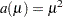and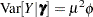. If your model calls for G-side random effects only (see the next section), the procedure models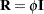, whereis the identity matrix. Table 40.15 identifies the distributions for which.